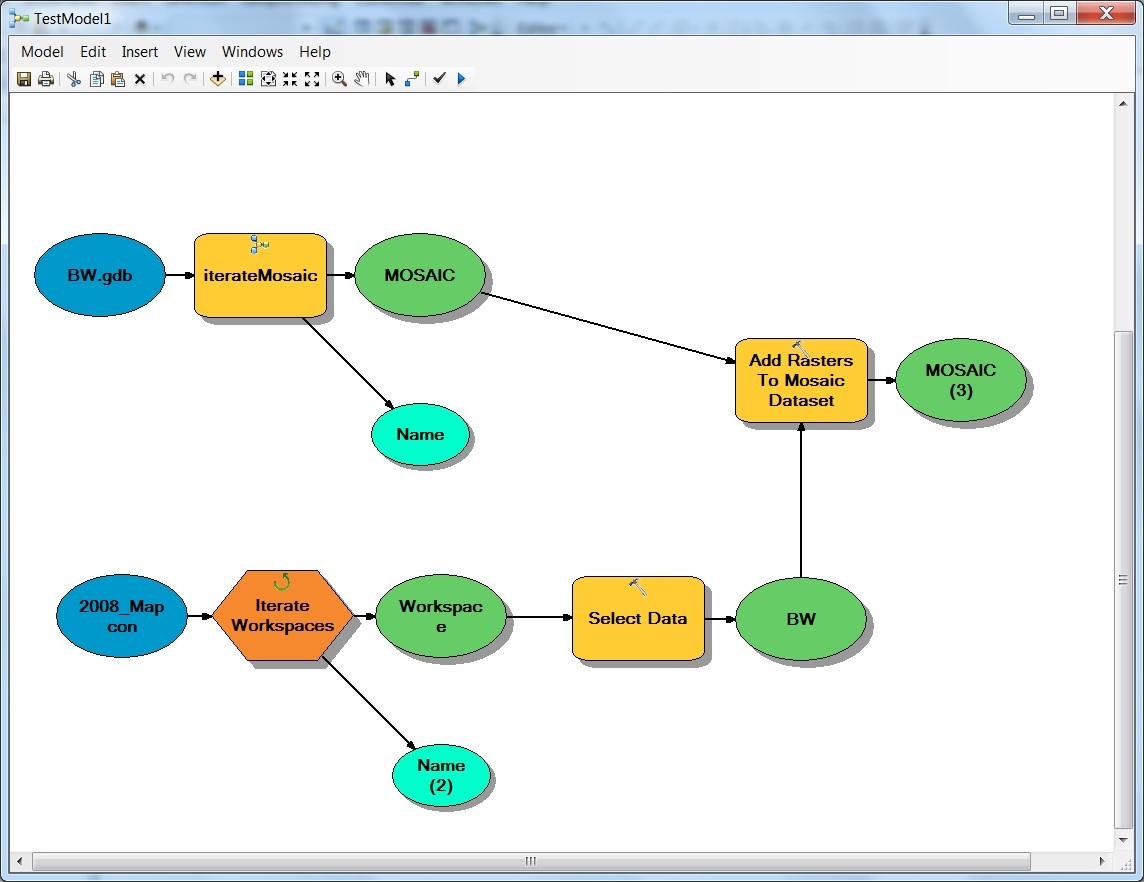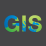# Iterate Dataset and Workspace to Add Rasters to Mosaic Dataset

1937
2
02-23-2017 05:47 PMby
New Contributor II

My raster mosaic datasets are in a file gdb ordered by block. For example Block_470 and Block_480.

My workspace has images ordered by block with subfolders with in each block. For example workspace Block_470 contains subfolders BW and RGB. This is the same for workspace Block_480. Meaning workspace Block_480 will also have subfolders BW and RGB.

I need to add the tiff files in workspace Block_470/BW to raster mosaic dataset Block_470 and the tiff files in workspace Block_480/BW will need to be added to raster mosaic dataset Block_480.

How can I iterate through so that the right tiff files in the subfolders go into the corresponding mosaic datasets?

I was thinking something like this:

``````import arcpy

workspaces = arcpy.ListWorkspaces("//nyx-galli/eGIS/Data/Raster/Imagery/Aerial/SGIC/2008_Mapcon/Block4*", "Folder")
datasets = arcpy.ListDatasets("D:/TestRasterMosaic/BW.gdb/Block_4*", "Mosaic")

for i in workspaces:
workspaces2 = arcpy.ListWorkspaces("", "Folder")‍‍‍‍‍‍‍``````

This did not work for me:1 Solution

Accepted SolutionsbyEsri Esteemed Contributor

Hi Amy,

Try something like the following:

``````import arcpy, os
from arcpy import env
env.workspace = r"D:\temp\python\userdata\images"

walk = arcpy.da.Walk(env.workspace)

for dirpath, dirnames, filenames in walk:
if 'BW' in dirpath:
# Path to mosaic dataset
mosaicDataset = r"D:\raster\MosaicDatasets.gdb" + "\\" + str(dirpath.split("\\")[-2])
# Add rasters from BW folder
2 RepliesbyEsri Esteemed Contributor

Hi Amy,

Try something like the following:

``````import arcpy, os
from arcpy import env
env.workspace = r"D:\temp\python\userdata\images"

walk = arcpy.da.Walk(env.workspace)

for dirpath, dirnames, filenames in walk:
if 'BW' in dirpath:
# Path to mosaic dataset
mosaicDataset = r"D:\raster\MosaicDatasets.gdb" + "\\" + str(dirpath.split("\\")[-2])
# Add rasters from BW folderby
New Contributor II

Hi Jake,

Thank you for the suggestion! arcpy.da.Walk() was definitely needed.

If this helps anyone else.

This is what I used to get it working:

``````import arcpy, os

basePath = r"\\nyx-galli\eGIS\Data\Raster\Imagery\Aerial\SGIC\2008_Mapcon"
db = os.path.join(basePath, r"BW.gdb")

walk = arcpy.da.Walk(basePath, datatype="RasterDataset", type="TIF")

for dirpath, dirnames, filenames in walk:
xPath = os.path.normpath(dirpath).split(os.sep)

if xPath[-1] == 'BW':
arcpy.env.workspace = dirpath
print dirpath

block = xPath[-2]

mosaicDataset = "{0}".format(block)
print mosaicDataset

if filenames: